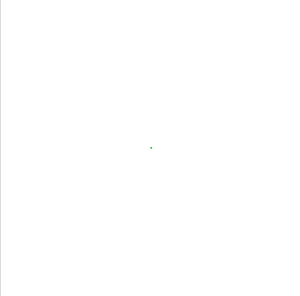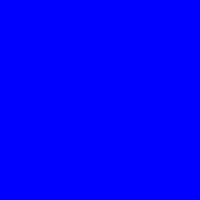GeeksforGeeks App
Open AppBrowser
Continue

# Wand color() function in Python

color() function draws a color on the image using current fill color, starting at specified position & method. Uses same arguments as color() method. Following are PAINT_METHOD_TYPES.

• ‘point’ alters a single pixel.
• ‘replace’ swaps on color for another. Threshold is influenced by fuzz.
• ‘floodfill’ fills area of a color influenced by fuzz.
• ‘filltoborder’ fills area of a color until border defined by border_color.
• ‘reset’ replaces the whole image to a single color.

Syntax :

`wand.drawing.color(x, y, method)`

Parameters :

Example #1:

## Python3

 `# Import required objects from wand modules``from` `wand.image ``import` `Image``from` `wand.drawing ``import` `Drawing``from` `wand.color ``import` `Color` `# generate object for wand.drawing``with Drawing() as draw:``    ``draw.fill_color ``=` `Color(``'green'``)``    ``draw.color(``100``, ``100``, ``'point'``)``    ``with Image(width ``=` `200``,``               ``height ``=` `200``,``               ``background ``=` `Color(``'white'``)) as img:``        ``# draw shape on image using draw() function``        ``draw.draw(img)``        ``img.save(filename ``=``'color.png'``)`

Output: A green pixel is visible at center of transparent image here is the zoomed image.Example #2: Filling color using flood-fill algorithm.

## Python3

 `# Import required objects from wand modules``from` `wand.image ``import` `Image``from` `wand.drawing ``import` `Drawing``from` `wand.color ``import` `Color` `# generate object for wand.drawing``with Drawing() as draw:``    ``draw.fill_color ``=` `Color(``'blue'``)``    ``draw.alpha(``10``, ``35``, ``'floodfill'``)``    ``with Image(width ``=` `200``,``               ``height ``=` `200``,``               ``background ``=` `Color(``'white'``)) as img:``        ``# draw shape on image using draw() function``        ``draw.draw(img)``        ``img.save(filename ``=``'color2.png'``)`

Output:My Personal Notes arrow_drop_up